# How to Calculate the Approximate Area of Irregular Shapes Using Simpson’s 1/3 Rule

• Author:
• Updated date:

Ray is a licensed engineer in the Philippines. He loves to write about mathematics and civil engineering.

## Introduction to Area Approximation

Are you having trouble solving areas of complicated and irregular shaped curve figures? If yes, this is the perfect article for you. There are a lot of methods and formulas used in approximating the area of irregularly shaped curves, just as shown in the figure below. Among these are Simpson’s Rule, the Trapezoidal Rule, and Durand’s Rule.

Trapezoidal Rule is an integration rule where you divide the total area of the irregular shaped figure into little trapezoids before evaluating the area under a specific curve. Durand’s Rule is a slightly more complicated but more precise integration rule than the trapezoidal rule. This method of area approximation uses the Newton-Cotes formula, which is an extremely useful and straightforward integration technique. Lastly, Simpson’s Rule gives the most accurate approximation as compared to the other two mentioned formulas. It is also important to note that the greater the value of n in Simpson’s Rule, the greater accuracy of area approximation.

## What Is Simpson’s 1/3 Rule?

Simpson’s Rule is named after the English mathematician Thomas Simpson who was from Leicestershire England. But for some reason, the formulas used in this method of area approximation were similar to Johannes Kepler’s formulas used over 100 years prior. That is the reason why many mathematicians call this method the Kepler’s Rule.

Simpson’s Rule is considered as a very diverse numerical integration technique. It is entirely based on the type of interpolation you will use. Simpson’s 1/3 Rule or Composite Simpson’s Rule is based upon a quadratic interpolation while Simpson’s 3/8 Rule is based upon a cubic interpolation. Among all methods of area approximation, Simpson’s 1/3 Rule gives the most accurate area because parabolas are used to approximate each part of the curve, and not rectangles or trapezoids.

Simpson's 1/3 Rule states that if y0, y1, y2,...,y3 (n is even) are the lengths of a series of parallel chords of uniform interval d, the area of the figure enclosed above is given approximately by the formula below. Note that if the figure ends with points, then take y0 = yn = 0.

## Problem 1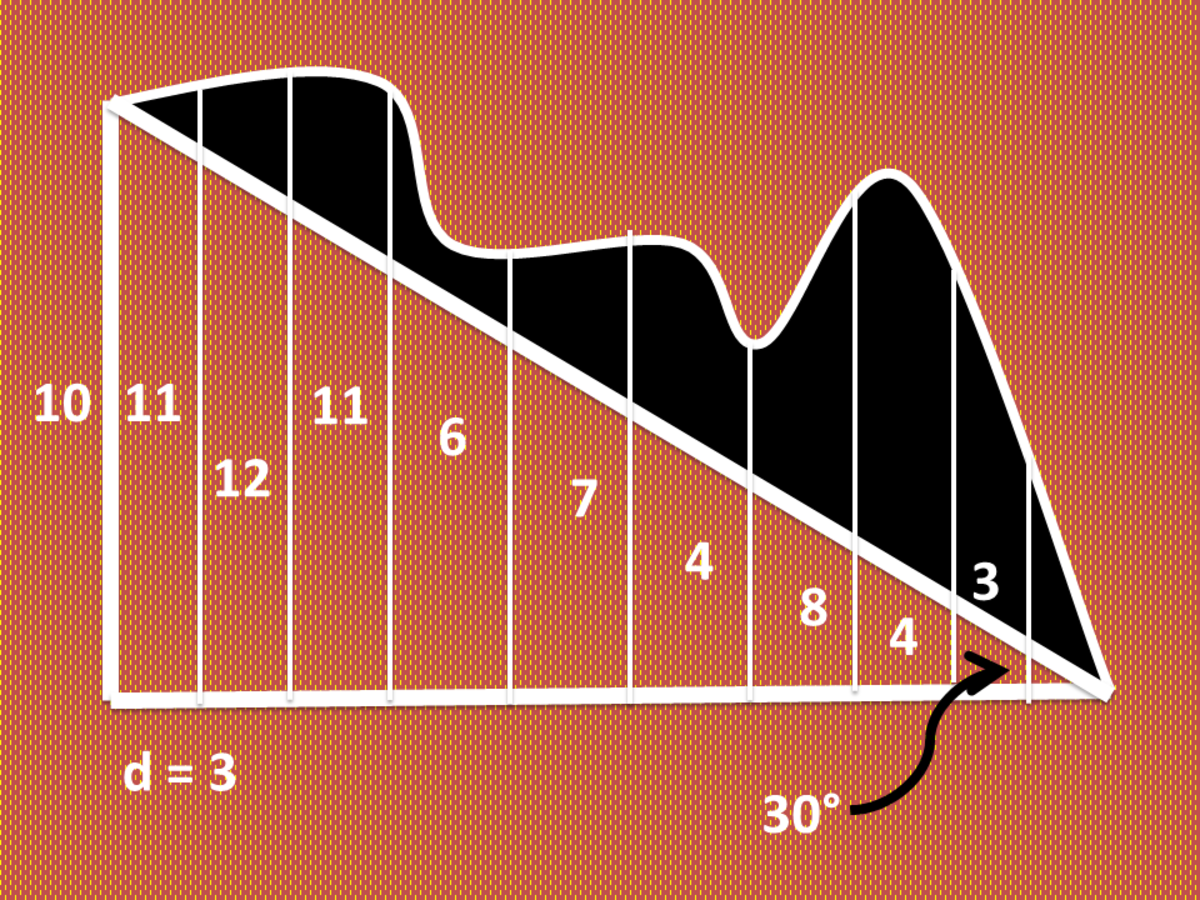Calculating the Area of Irregular Shapes Using Simpson's 1/3 RuleJohn Ray Cuevas

### Solution

a. Given the value of n = 10 of the irregularly shaped figure, identify the height values from y0 to y10. Create a table and list all the height values from left to right for a more organized solution.

Calculating the Approximate Area of Irregular Shapes Using Simpson’s 1/3 Rule

Variable (y)Height Value

y0

10

y1

11

y2

12

y3

11

y4

6

y5

7

y6

4

y7

8

y8

4

y9

3

y10

0

b. The given value of the uniform interval is d = 0.75. Substitute the height values (y) in the given Simpson's rule equation. The resulting answer is the approximate area of the given shape above.

A = (1/3)(d) [(y0 + yn) + 4(y1 + y3 + ... + yn-1) + 2(y2 + y4 + ... + yn-2)]

A = (1/3)(3) [(10 + 0) + 4(11 + 11 + 7 + 8 + 3) + 2(12 + 6 + 4 + 4)]

A = 222 square units

c. Find the area of the right triangle formed from the irregular shape. Given a height of 10 units and an angle of 30°, find the length of adjacent sides and compute for the area of the right triangle using Scissors formula or Heron's formula.

Length = 10 / tan (30°)

Scroll to Continue

Length = 17.32 units

Hypotenuse = 10 / sin (30°)

Hypotenuse = 20 units

Semi-Perimeter (s) = (10 + 20 + 17.32) / 2

Semi-Perimeter (s) = 23. 66 units

Area (A) = √s (s - a) (s - b) (s - c)

Area (A) = √23.66 (23.66 - 10) (23.66 - 20) (23.66 - 17.32)

Area (A) = 86.6 square units

d. Subtract the area of the right triangle from the area of the whole irregular figure.

Shaded Area (S) = Total Area - Triangular Area

Shaded Area (S) = 222 - 86.6

Shaded Area (S) = 135.4 square units

Final Answer: The approximate area of the irregular figure above is 135.4 square units.

## Problem 2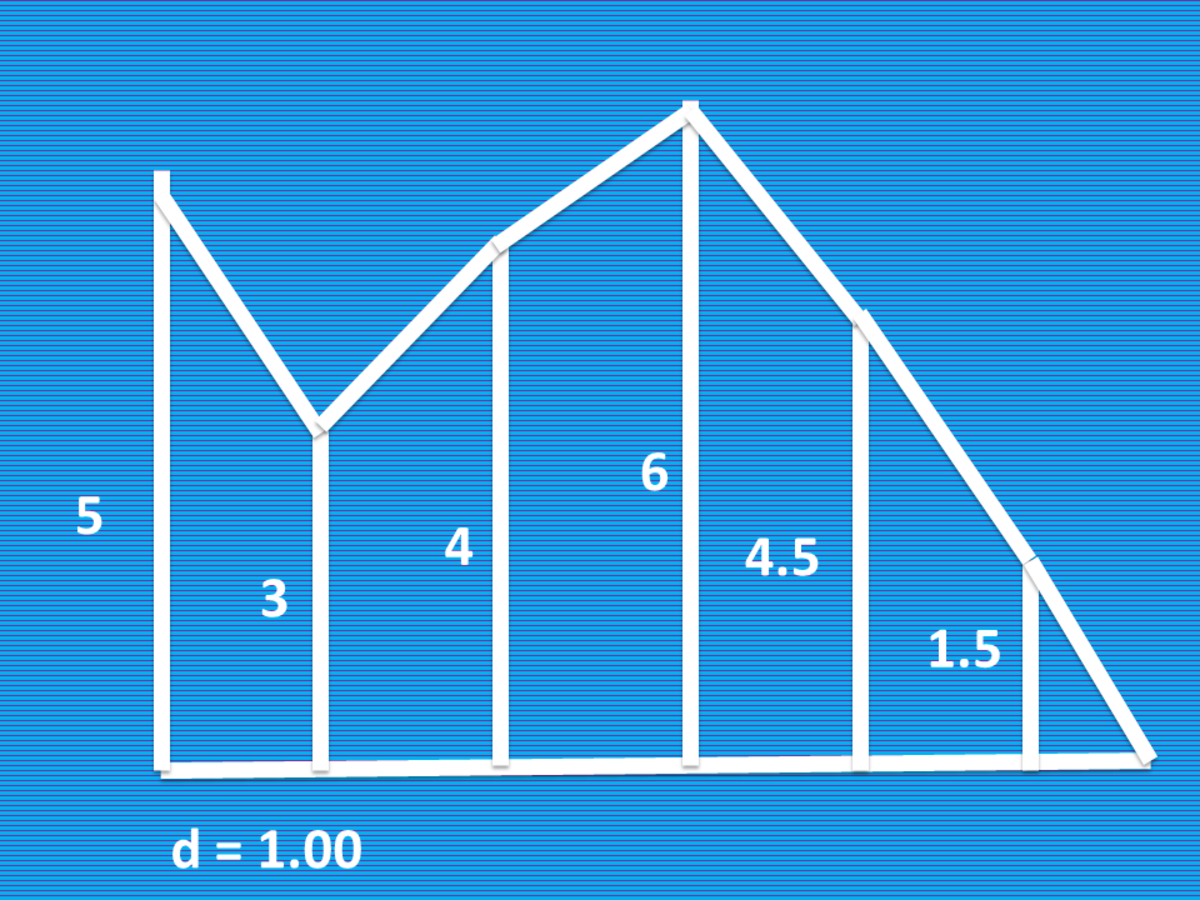Calculating the Area of Irregular Shapes Using Simpson's 1/3 RuleJohn Ray Cuevas

### Solution

a. Given the value of n = 6 of the irregularly shaped figure, identify the height values from y0 to y6. Create a table and list all the height values from left to right for a more organized solution.

Calculating the Approximate Area of Irregular Shapes Using Simpson’s 1/3 Rule

Variable (y)Height Value

y0

5

y1

3

y2

4

y3

6

y4

4.5

y5

1.5

y6

0

b. The given value of the uniform interval is d = 1.00. Substitute the height values (y) in the given Simpson's rule equation. The resulting answer is the approximate area of the given shape above.

A = (1/3)(d) [(y0 + yn) + 4(y1 + y3 + ... + yn-1) + 2(y2 + y4 + ... + yn-2)]

A = (1/3)(1.00) [(5 + 0) + 4(3 + 6 + 1.5) + 2(4 + 4.5)]

A = 21.33 square units

Final Answer: The approximate area of the irregular figure above is 21.33 square units.

## Problem 3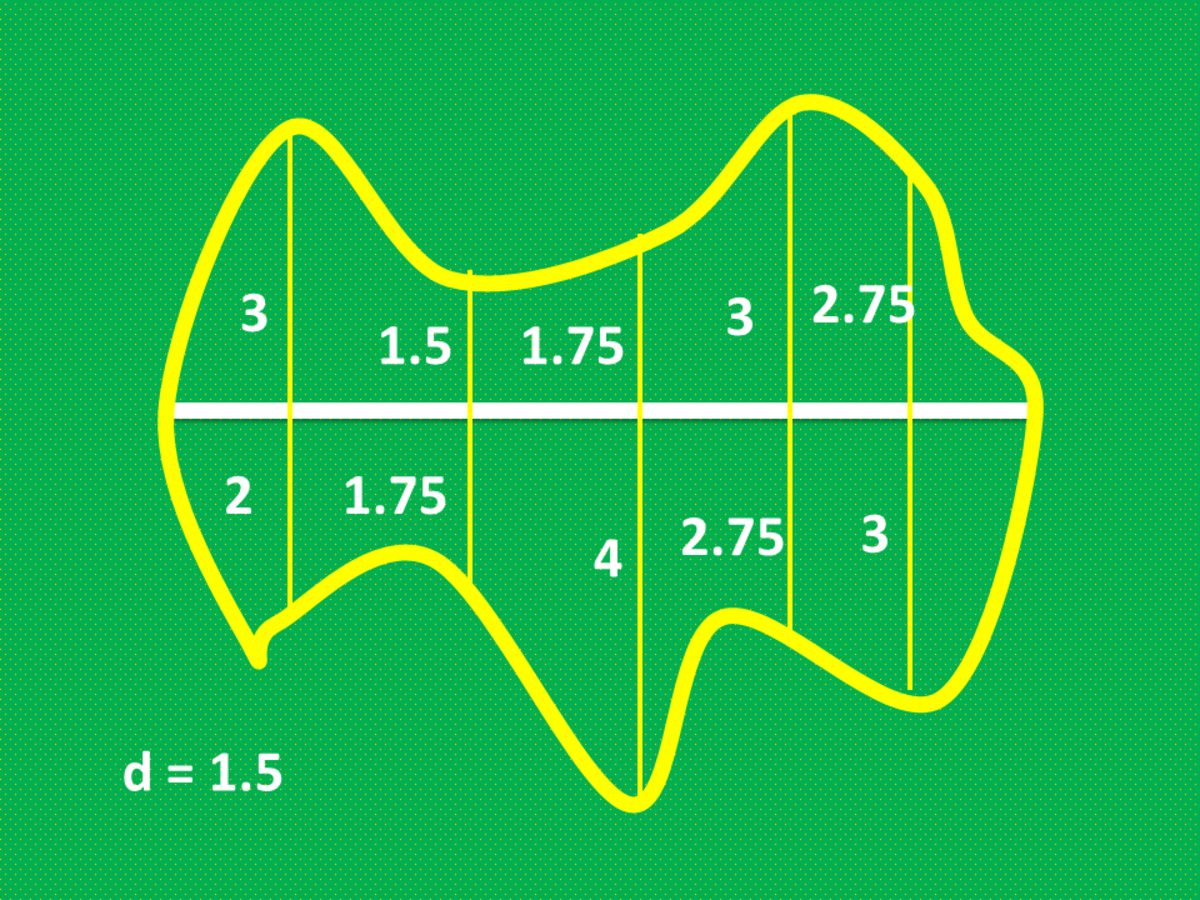Calculating the Area of Irregular Shapes Using Simpson's 1/3 RuleJohn Ray Cuevas

### Solution

a. Given the value of n = 6 of the irregularly shaped figure, identify the height values from y0 to y6. Create a table and list all the height values from left to right for a more organized solution.

Calculating the Approximate Area of Irregular Shapes Using Simpson’s 1/3 Rule

Variable (y)Upper ValueLower ValueHeight Value (Sum)

y0

0

0

0

y1

3

2

5

y2

1.5

1.75

3.25

y3

1.75

4

5.75

y4

3

2.75

5.75

y5

2.75

3

5.75

y6

0

0

0

b. The given value of the uniform interval is d = 1.50. Substitute the height values (y) in the given Simpson's rule equation. The resulting answer is the approximate area of the given shape above.

A = (1/3)(d) [(y0 + yn) + 4(y1 + y3 + ... + yn-1) + 2(y2 + y4 + ... + yn-2)]

A = (1/3)(1.50) [(0 + 0) + 4(5 + 5.75 + 5.75) + 2(3.25 + 5.75)]

A = 42 square units

Final Answer: The approximate area of the irregular shape above is 42 square units.

## Problem 4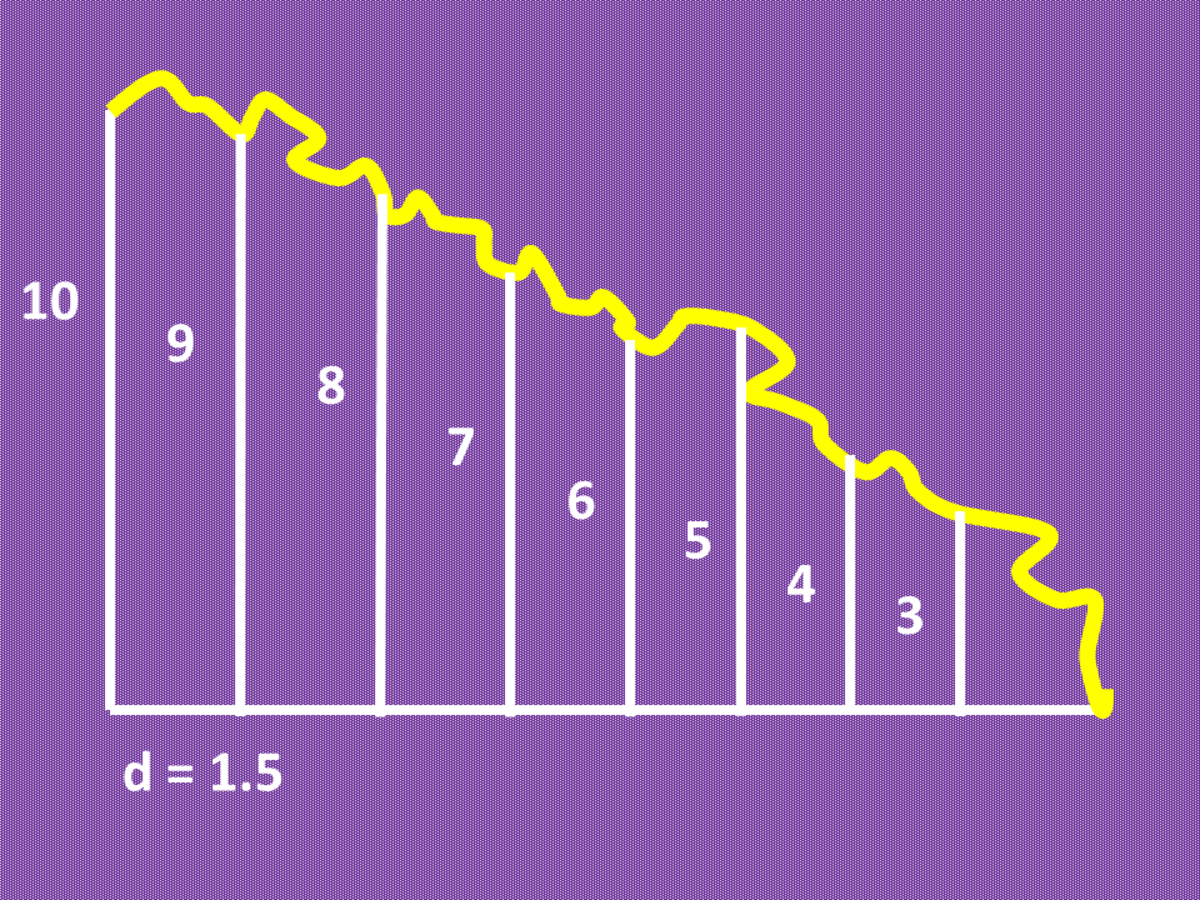Calculating the Area of Irregular Shapes Using Simpson's 1/3 RuleJohn Ray Cuevas

### Solution

a. Given the value of n = 8 of the irregularly shaped figure, identify the height values from y0 to y8. Create a table and list all the height values from left to right for a more organized solution.

Calculating the Approximate Area of Irregular Shapes Using Simpson’s 1/3 Rule

Variable (y)Height Value

y0

10

y1

9

y2

8

y3

7

y4

6

y5

5

y6

4

y7

3

y8

0

b. The given value of the uniform interval is d = 1.50. Substitute the height values (y) in the given Simpson's rule equation. The resulting answer is the approximate area of the given shape above.

A = (1/3)(d) [(y0 + yn) + 4(y1 + y3 + ... + yn-1) + 2(y2 + y4 + ... + yn-2)]

A = (1/3)(1.50) [(10 + 0) + 4(9 + 7 + 5 +3) + 2(8 + 6 +4)]

A = 71 square units

Final Answer: The approximate area of the irregular shape above is 71 square units.

## Problem 5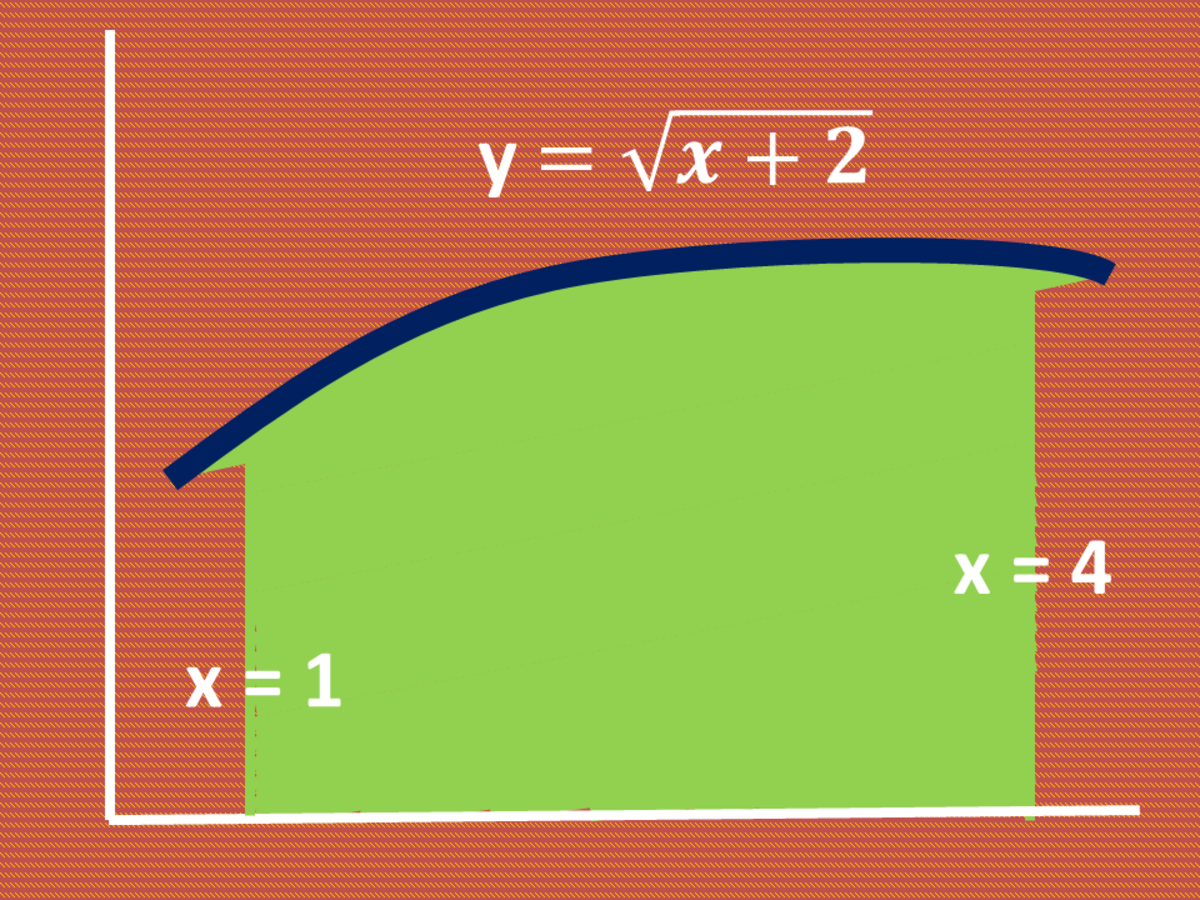Calculating the Area of Irregular Shapes Using Simpson's 1/3 RuleJohn Ray Cuevas

### Solution

a. Given the equation of the irregular curve, identify the height values from y0 to y8 by substituting each value of x to solve for the corresponding value of y. Create a table and list all the height values from left to right for a more organized solution. Use an interval of 0.5.

Calculating the Approximate Area of Irregular Shapes Using Simpson’s 1/3 Rule

Variable (y)X-ValueHeight Value

y0

1.0

1.732050808

y1

1.5

1.870828693

y2

2.0

2.0000000

y3

2.5

2.121320344

y4

3.0

2.236067977

y5

3.5

2.34520788

y6

4.0

2.449489743

b. Use the uniform interval d = 0.50. Substitute the height values (y) in the given Simpson's rule equation. The resulting answer is the approximate area of the given shape above.

A = (1/3)(d) [(y0 + yn) + 4(y1 + y3 + ... + yn-1) + 2(y2 + y4 + ... + yn-2)]

A = (1/3)(0.50) [(1.73 + 2.45) + 4(1.87 + 2.12 + 2.35) + 2(2 + 2.23)]

A = 6.33 square units

Final Answer: The approximate area of the irregular shape above is 6.33 square units.

## Problem 6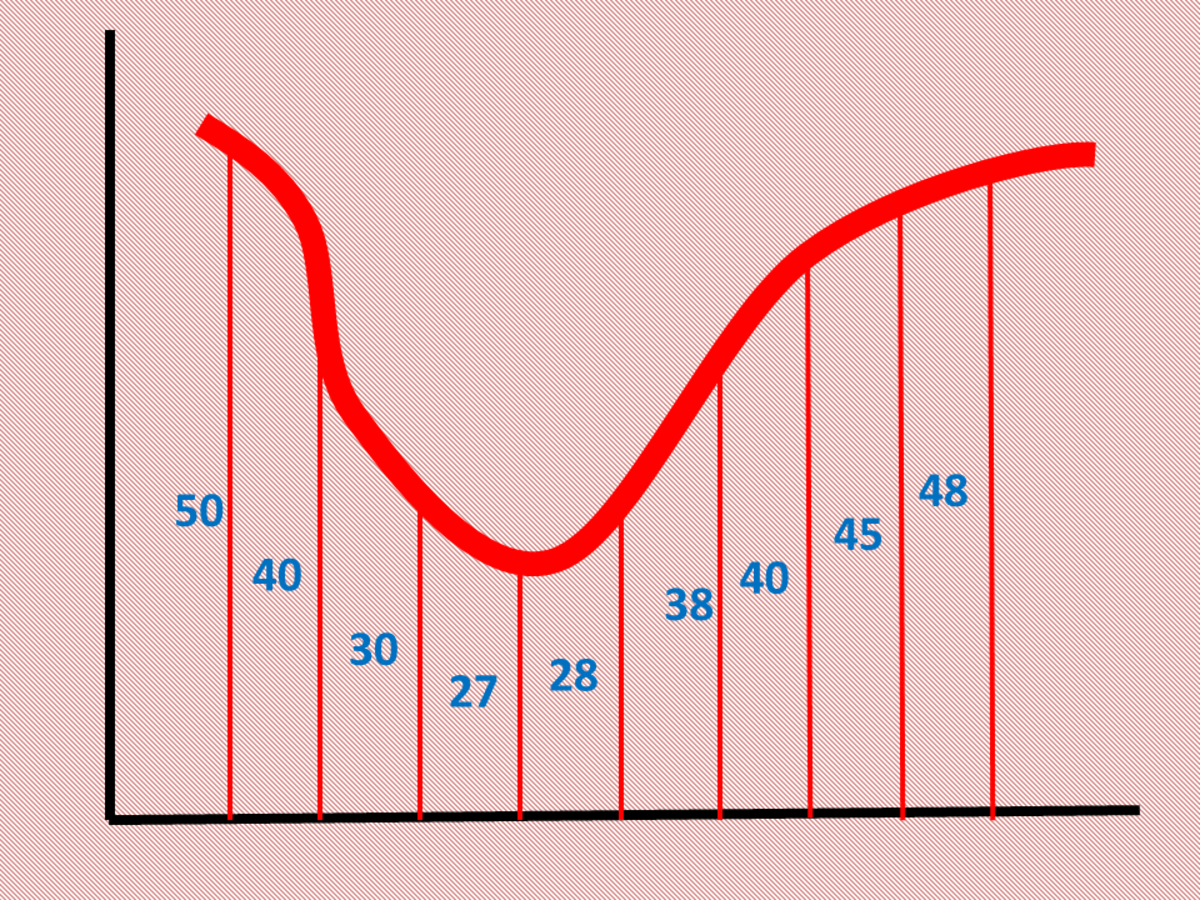Calculating the Area of Irregular Shapes Using Simpson's 1/3 RuleJohn Ray Cuevas

### Solution

a. Given the value of n = 8 of the irregularly shaped figure, identify the height values from y0 to y8. Create a table and list all the height values from left to right for a more organized solution.

Calculating the Approximate Area of Irregular Shapes Using Simpson’s 1/3 Rule

Variable (y)Height Value

y0

50

y1

40

y2

30

y3

27

y4

28

y5

38

y6

40

y7

45

y8

48

b. The given value of the uniform interval is d = 5.50. Substitute the height values (y) in the given Simpson's rule equation. The resulting answer is the approximate area of the given shape above.

A = (1/3)(d) [(y0 + yn) + 4(y1 + y3 + ... + yn-1) + 2(y2 + y4 + ... + yn-2)]

A = (1/3)(5.50) [(50 + 48) + 4(40 + 27 +38 +45) + 2(30 +28 + 40)]

A = 1639 square units

Final Answer: The approximate area of the irregular shape above is 1639 square units.